Special Binomial FormsCustom SearchSPECIAL BINOMIAL FORMS Special cases involving binomial expressions are frequently encountered. All such expressions may be factored by reference to general formulas, but these formulas are beyond the scope of this course. For our purposes, analysis of some special cases will be sufficient. Even Exponents When the exponents on both terms of the binomial are even, the expression may be treated as the sums or difference of two squares. For example, x6 - y6 can be rewritten as (x3)2 - (y3)2 which results in the following factored . .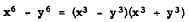In general, a binomial with even exponents has the form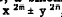, since all even numbers have 2 as a factor. If the connecting sign is positive, the expression may not be factorable; for example, x2 + y2, x4 + y4, and x6 + y6 are all nonfactorable binomials. If the .connecting sign is negative, a binomial with even exponents is factorable as follows: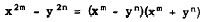A special case which is particularly important because it occurs so often is the binomial which has the numeral 1 as one of its terms. For example, x4 - 1 is factorable as the difference of two squares, as follows:Odd Exponents Two special cases involving odd exponents are of particular importance. These are the sum of two cubes and the difference of two cubes. Examples of the sum and difference of two cubes, showing their factored forms, are as follows: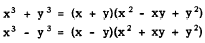Notice that each of these factored forms involves a first degree binomial factor ((x + y) in the first case and (x - y) in the second). The connecting sign in the first degree binomial factor corresponds to the connecting sign in the. original unfactored binomial. We are now in a position to give the completely factored form of x6 - y6, as follows: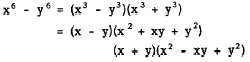In general, (x + y) is a factor of (xn + yn) if n is odd. If n is even, (xn + yn) is not factorable unless it can be expressed as the sum of two cubes. When the connecting sign is negative, the binomial is always factorable if n is a whole number greater than 1. That is, (x - y) is a factor of (xn - y n) for both odd and even values of n. The special case in which one of the terms of the binomial is the numeral 1 occurs frequently. An example of this is x3 + 1, which is factorable as the sum of two cubes, as follows: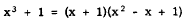In a similar manner, 1 + x6 can be treated as the sum of two cubes and factored as follows: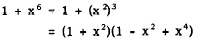Practice problems. In each of the following problems, factor completely: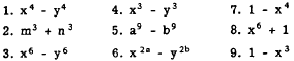Answers: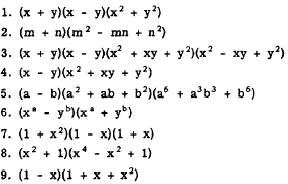Integrated Publishing, Inc. - A (SDVOSB) Service Disabled Veteran Owned Small Business